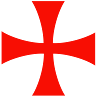cancel
Showing results for
Did you mean:

Head's Up! Site migration is underway. Phase 2: migrate recent content

## Get all nodes linked by a specific relationshipI have created a vehicle make called `Toyota` and also created a new node store my vehicle models.

CREATE (Make:make{name: "Toyota"})
CREATE (Model:model {name: "Camry"})
CREATE (Make)-[r:MODEL_OF]->(Model)
RETURN Make, Model

I added two more vehicle models

CREATE (Model:model {name: "Vitz"})
CREATE (Model:model {name: "Corolla"})

and linked the two models to `Toyota`

MATCH (a:make), (b:model) WHERE a.name = "Toyota" AND b.name = "Vitz"
CREATE (a)-[r: MODEL_OF]->(b)
RETURN a,b

MATCH (a:make), (b:model) WHERE a.name = "Toyota" AND b.name = "Corolla"
CREATE (a)-[r: MODEL_OF]->(b)
RETURN a,b

How can i return all models of make `Toyota` that have the relationship `MODEL_OF`?

1 ACCEPTED SOLUTIONNinja

Try this:

``````match(n:make{name:"Toyota"})-[:MODEL_OF]->(m:model)
return m.name as model``````

Just a note, I would have the relationship in your model directed the opposite way, i.e. a model is-a model of a make.3 REPLIES 3Ninja

Try this:

``````match(n:make{name:"Toyota"})-[:MODEL_OF]->(m:model)
return m.name as model``````

Just a note, I would have the relationship in your model directed the opposite way, i.e. a model is-a model of a make.I have reversed the relationshipsCREATE (Make:make{name: "Toyota"})
CREATE (Model:model {name: "Camry"})
CREATE (Model:model {name: "Vitz"})
CREATE (Model:model {name: "Corolla"})

Relationships

MATCH (a:model), (b:make) WHERE a.name = "Vitz" AND b.name = "Toyota"
CREATE (a)-[r: MODEL_OF]->(b)
RETURN a,b

MATCH (a:model), (b:make) WHERE a.name = "Corolla" AND b.name = "Toyota"
CREATE (a)-[r: MODEL_OF]->(b)
RETURN a,b

MATCH (a:model), (b:make) WHERE a.name = "Camry" AND b.name = "Toyota"
CREATE (a)-[r: MODEL_OF]->(b)
RETURN a,b

How can i modify your query?Solved it like this

MATCH (Make:make {name: "Toyota"})<-[r:MODEL_OF]-(n)
RETURN n.name as ModelNodes 2022NODES 2022, Neo4j Online Education Summit

All the sessions of the conference are now available online

Neo4j Resources